# Mean State

Period Mean (original grids) [Watt m-2]
Model Period Mean (intersection) [Watt m-2]
Model Period Mean (complement) [Watt m-2]
Benchmark Period Mean (intersection) [Watt m-2]
Benchmark Period Mean (complement) [Watt m-2]
Bias [Watt m-2]
RMSE [Watt m-2]
Phase Shift [months]
Bias Score 
RMSE Score 
Seasonal Cycle Score 
Spatial Distribution Score 
Interannual Variability Score 
Overall Score 
Benchmark [-] 179.
CRUNCEPv7 [-] 191. 191. 0.00 177. 216. 14.0 24.2 1.11 0.44 0.45 0.85 0.95 0.52 0.61
GSWP3v1 [-] 183. 183. 0.00 177. 216. 5.83 16.7 0.533 0.63 0.52 0.93 0.94 0.73 0.71
WATCH [-] 140. 140. 0.00 177. 217. -36.3 42.4 1.57 0.25 0.41 0.76 0.83 0.77 0.57
Period Mean (original grids) [Watt m-2]
Model Period Mean (intersection) [Watt m-2]
Model Period Mean (complement) [Watt m-2]
Benchmark Period Mean (intersection) [Watt m-2]
Benchmark Period Mean (complement) [Watt m-2]
Bias [Watt m-2]
RMSE [Watt m-2]
Phase Shift [months]
Bias Score 
RMSE Score 
Seasonal Cycle Score 
Spatial Distribution Score 
Interannual Variability Score 
Overall Score 
Benchmark [-] 189.
CRUNCEPv7 [-] 198. 198. 0.00 188. 224. 9.50 22.8 1.64 0.58 0.46 0.76 0.90 0.67 0.64
GSWP3v1 [-] 195. 195. 0.00 188. 224. 6.89 16.6 0.458 0.66 0.53 0.95 1.0 0.78 0.74
WATCH [-] 159. 160. 0.00 188. 224. -27.9 33.6 0.577 0.34 0.49 0.93 0.85 0.64 0.62
Period Mean (original grids) [Watt m-2]
Model Period Mean (intersection) [Watt m-2]
Model Period Mean (complement) [Watt m-2]
Benchmark Period Mean (intersection) [Watt m-2]
Benchmark Period Mean (complement) [Watt m-2]
Bias [Watt m-2]
RMSE [Watt m-2]
Phase Shift [months]
Bias Score 
RMSE Score 
Seasonal Cycle Score 
Spatial Distribution Score 
Interannual Variability Score 
Overall Score 
Benchmark [-] 194.
CRUNCEPv7 [-] 194. 194. 0.00 192. 221. 2.03 22.6 1.19 0.69 0.50 0.84 0.75 0.53 0.64
GSWP3v1 [-] 197. 197. 0.00 192. 221. 3.85 18.7 0.529 0.72 0.56 0.93 0.96 0.67 0.73
WATCH [-] 180. 180. 0.00 192. 221. -12.3 24.3 0.642 0.58 0.55 0.92 0.97 0.68 0.71
Period Mean (original grids) [Watt m-2]
Model Period Mean (intersection) [Watt m-2]
Model Period Mean (complement) [Watt m-2]
Benchmark Period Mean (intersection) [Watt m-2]
Benchmark Period Mean (complement) [Watt m-2]
Bias [Watt m-2]
RMSE [Watt m-2]
Phase Shift [months]
Bias Score 
RMSE Score 
Seasonal Cycle Score 
Spatial Distribution Score 
Interannual Variability Score 
Overall Score 
Benchmark [-] 81.3
CRUNCEPv7 [-] 74.9 75.0 0.00 80.9 86.2 -7.45 22.7 0.352 0.88 0.73 0.98 0.87 0.61 0.80
GSWP3v1 [-] 72.8 72.9 0.00 80.9 86.2 -9.00 20.1 0.218 0.87 0.75 0.99 0.92 0.70 0.83
WATCH [-] 65.2 65.3 0.00 81.6 87.0 -16.4 23.2 0.255 0.79 0.76 0.98 0.97 0.69 0.83
Period Mean (original grids) [Watt m-2]
Model Period Mean (intersection) [Watt m-2]
Model Period Mean (complement) [Watt m-2]
Benchmark Period Mean (intersection) [Watt m-2]
Benchmark Period Mean (complement) [Watt m-2]
Bias [Watt m-2]
RMSE [Watt m-2]
Phase Shift [months]
Bias Score 
RMSE Score 
Seasonal Cycle Score 
Spatial Distribution Score 
Interannual Variability Score 
Overall Score 
Benchmark [-] 189.
CRUNCEPv7 [-] 186. 186. 0.00 183. 210. 5.12 25.9 1.53 0.49 0.41 0.77 0.55 0.47 0.52
GSWP3v1 [-] 177. 177. 0.00 183. 210. -2.07 20.5 0.665 0.61 0.46 0.91 0.73 0.71 0.65
WATCH [-] 142. 141. 0.00 184. 210. -34.4 41.1 1.46 0.29 0.39 0.78 0.91 0.76 0.58
Period Mean (original grids) [Watt m-2]
Model Period Mean (intersection) [Watt m-2]
Model Period Mean (complement) [Watt m-2]
Benchmark Period Mean (intersection) [Watt m-2]
Benchmark Period Mean (complement) [Watt m-2]
Bias [Watt m-2]
RMSE [Watt m-2]
Phase Shift [months]
Bias Score 
RMSE Score 
Seasonal Cycle Score 
Spatial Distribution Score 
Interannual Variability Score 
Overall Score 
Benchmark [-] 105.
CRUNCEPv7 [-] 108. 108. 0.00 104. 127. 4.10 20.9 0.174 0.90 0.76 0.99 0.99 0.61 0.83
GSWP3v1 [-] 100. 100. 0.00 104. 127. -3.81 16.7 0.0856 0.93 0.80 0.99 1.0 0.64 0.86
WATCH [-] 87.3 87.3 0.00 105. 128. -17.6 23.0 0.259 0.78 0.81 0.98 0.97 0.67 0.84
Period Mean (original grids) [Watt m-2]
Model Period Mean (intersection) [Watt m-2]
Model Period Mean (complement) [Watt m-2]
Benchmark Period Mean (intersection) [Watt m-2]
Benchmark Period Mean (complement) [Watt m-2]
Bias [Watt m-2]
RMSE [Watt m-2]
Phase Shift [months]
Bias Score 
RMSE Score 
Seasonal Cycle Score 
Spatial Distribution Score 
Interannual Variability Score 
Overall Score 
Benchmark [-] 140.
CRUNCEPv7 [-] 149. 148. 0.00 139. 154. 8.93 26.1 0.567 0.75 0.69 0.95 0.97 0.64 0.78
GSWP3v1 [-] 136. 135. 0.00 139. 154. -3.72 16.9 0.242 0.89 0.74 0.98 1.0 0.62 0.83
WATCH [-] 132. 132. 0.00 141. 155. -8.59 22.2 0.424 0.80 0.72 0.96 0.98 0.70 0.81
Period Mean (original grids) [Watt m-2]
Model Period Mean (intersection) [Watt m-2]
Model Period Mean (complement) [Watt m-2]
Benchmark Period Mean (intersection) [Watt m-2]
Benchmark Period Mean (complement) [Watt m-2]
Bias [Watt m-2]
RMSE [Watt m-2]
Phase Shift [months]
Bias Score 
RMSE Score 
Seasonal Cycle Score 
Spatial Distribution Score 
Interannual Variability Score 
Overall Score 
Benchmark [-] 180.
CRUNCEPv7 [-] 194. 194. 0.00 178. 210. 15.4 31.1 0.410 0.54 0.54 0.97 0.96 0.53 0.68
GSWP3v1 [-] 175. 175. 0.00 178. 210. -1.80 18.7 0.253 0.81 0.62 0.98 0.92 0.63 0.76
WATCH [-] 159. 159. 0.00 179. 210. -18.7 30.2 0.434 0.58 0.57 0.97 0.95 0.66 0.72
Period Mean (original grids) [Watt m-2]
Model Period Mean (intersection) [Watt m-2]
Model Period Mean (complement) [Watt m-2]
Benchmark Period Mean (intersection) [Watt m-2]
Benchmark Period Mean (complement) [Watt m-2]
Bias [Watt m-2]
RMSE [Watt m-2]
Phase Shift [months]
Bias Score 
RMSE Score 
Seasonal Cycle Score 
Spatial Distribution Score 
Interannual Variability Score 
Overall Score 
Benchmark [-] 74.6
CRUNCEPv7 [-] 68.2 68.6 0.00 74.6 74.8 -7.24 21.8 0.337 0.87 0.72 0.98 0.84 0.62 0.79
GSWP3v1 [-] 64.0 64.4 0.00 74.6 74.8 -11.2 19.0 0.311 0.84 0.74 0.98 0.93 0.67 0.82
WATCH [-] 51.9 52.2 0.00 75.0 75.5 -23.3 25.8 0.363 0.70 0.74 0.98 0.98 0.67 0.80
Period Mean (original grids) [Watt m-2]
Model Period Mean (intersection) [Watt m-2]
Model Period Mean (complement) [Watt m-2]
Benchmark Period Mean (intersection) [Watt m-2]
Benchmark Period Mean (complement) [Watt m-2]
Bias [Watt m-2]
RMSE [Watt m-2]
Phase Shift [months]
Bias Score 
RMSE Score 
Seasonal Cycle Score 
Spatial Distribution Score 
Interannual Variability Score 
Overall Score 
Benchmark [-] 193.
CRUNCEPv7 [-] 194. 194. 0.00 193. 202. -0.170 20.8 0.537 0.82 0.68 0.96 0.95 0.59 0.78
GSWP3v1 [-] 192. 192. 0.00 193. 202. -1.99 18.1 0.222 0.85 0.70 0.98 1.0 0.61 0.81
WATCH [-] 197. 197. 0.00 194. 202. 0.952 20.2 0.346 0.81 0.70 0.97 1.0 0.76 0.82
Period Mean (original grids) [Watt m-2]
Model Period Mean (intersection) [Watt m-2]
Model Period Mean (complement) [Watt m-2]
Benchmark Period Mean (intersection) [Watt m-2]
Benchmark Period Mean (complement) [Watt m-2]
Bias [Watt m-2]
RMSE [Watt m-2]
Phase Shift [months]
Bias Score 
RMSE Score 
Seasonal Cycle Score 
Spatial Distribution Score 
Interannual Variability Score 
Overall Score 
Benchmark [-] 120.
CRUNCEPv7 [-] 131. 131. 0.00 117. 151. 14.4 27.6 0.295 0.74 0.75 0.98 1.0 0.64 0.81
GSWP3v1 [-] 113. 113. 0.00 117. 151. -1.95 16.4 0.358 0.91 0.80 0.98 1.0 0.62 0.85
WATCH [-] 95.4 95.5 0.00 117. 151. -18.2 23.2 0.240 0.77 0.81 0.98 0.99 0.69 0.84
Period Mean (original grids) [Watt m-2]
Model Period Mean (intersection) [Watt m-2]
Model Period Mean (complement) [Watt m-2]
Benchmark Period Mean (intersection) [Watt m-2]
Benchmark Period Mean (complement) [Watt m-2]
Bias [Watt m-2]
RMSE [Watt m-2]
Phase Shift [months]
Bias Score 
RMSE Score 
Seasonal Cycle Score 
Spatial Distribution Score 
Interannual Variability Score 
Overall Score 
Benchmark [-] 168.
CRUNCEPv7 [-] 143. 143. 0.00 143. 180. -0.0159 23.9 0.610 0.71 0.63 0.93 0.96 0.55 0.74
GSWP3v1 [-] 137. 137. 0.00 143. 180. -5.85 18.9 0.278 0.79 0.67 0.97 0.97 0.62 0.78
WATCH [-] 126. 126. 0.00 144. 180. -17.4 26.5 0.473 0.64 0.65 0.95 0.98 0.66 0.76
Period Mean (original grids) [Watt m-2]
Model Period Mean (intersection) [Watt m-2]
Model Period Mean (complement) [Watt m-2]
Benchmark Period Mean (intersection) [Watt m-2]
Benchmark Period Mean (complement) [Watt m-2]
Bias [Watt m-2]
RMSE [Watt m-2]
Phase Shift [months]
Bias Score 
RMSE Score 
Seasonal Cycle Score 
Spatial Distribution Score 
Interannual Variability Score 
Overall Score 
Benchmark [-] 175.
CRUNCEPv7 [-] 168. 168. 0.00 173. 224. -4.48 21.6 0.402 0.71 0.70 0.96 1.0 0.59 0.77
GSWP3v1 [-] 162. 162. 0.00 173. 224. -10.2 19.7 0.305 0.72 0.73 0.97 0.99 0.58 0.79
WATCH [-] 147. 147. 0.00 174. 225. -25.4 30.3 0.397 0.55 0.71 0.97 1.0 0.55 0.75
Period Mean (original grids) [Watt m-2]
Model Period Mean (intersection) [Watt m-2]
Model Period Mean (complement) [Watt m-2]
Benchmark Period Mean (intersection) [Watt m-2]
Benchmark Period Mean (complement) [Watt m-2]
Bias [Watt m-2]
RMSE [Watt m-2]
Phase Shift [months]
Bias Score 
RMSE Score 
Seasonal Cycle Score 
Spatial Distribution Score 
Interannual Variability Score 
Overall Score 
Benchmark [-] 80.0
CRUNCEPv7 [-] 75.4 75.2 0.00 79.8 82.9 -6.91 22.0 0.127 0.85 0.73 0.99 0.79 0.62 0.79
GSWP3v1 [-] 70.5 70.4 0.00 79.8 82.9 -10.9 19.6 0.0911 0.84 0.76 0.99 0.89 0.72 0.83
WATCH [-] 66.1 65.9 0.00 80.2 83.5 -15.2 22.2 0.132 0.80 0.76 0.99 0.94 0.75 0.83
Period Mean (original grids) [Watt m-2]
Model Period Mean (intersection) [Watt m-2]
Model Period Mean (complement) [Watt m-2]
Benchmark Period Mean (intersection) [Watt m-2]
Benchmark Period Mean (complement) [Watt m-2]
Bias [Watt m-2]
RMSE [Watt m-2]
Phase Shift [months]
Bias Score 
RMSE Score 
Seasonal Cycle Score 
Spatial Distribution Score 
Interannual Variability Score 
Overall Score 
Benchmark [-] 205.
CRUNCEPv7 [-] 189. 189. 0.00 200. 227. -9.36 28.6 0.810 0.61 0.59 0.92 0.63 0.53 0.64
GSWP3v1 [-] 192. 192. 0.00 200. 227. -6.23 20.2 0.250 0.77 0.64 0.97 0.89 0.63 0.76
WATCH [-] 181. 181. 0.00 199. 226. -16.8 30.5 0.463 0.57 0.61 0.95 0.96 0.68 0.73
Period Mean (original grids) [Watt m-2]
Model Period Mean (intersection) [Watt m-2]
Model Period Mean (complement) [Watt m-2]
Benchmark Period Mean (intersection) [Watt m-2]
Benchmark Period Mean (complement) [Watt m-2]
Bias [Watt m-2]
RMSE [Watt m-2]
Phase Shift [months]
Bias Score 
RMSE Score 
Seasonal Cycle Score 
Spatial Distribution Score 
Interannual Variability Score 
Overall Score 
Benchmark [-] 153.
CRUNCEPv7 [-] 156. 156. 0.00 151. 194. 6.08 19.4 0.518 0.85 0.74 0.97 1.0 0.55 0.81
GSWP3v1 [-] 144. 144. 0.00 151. 194. -5.31 16.1 0.154 0.91 0.77 0.99 1.0 0.62 0.84
WATCH [-] 146. 145. 0.00 149. 193. -2.75 16.5 0.353 0.91 0.77 0.98 1.0 0.75 0.86
Period Mean (original grids) [Watt m-2]
Model Period Mean (intersection) [Watt m-2]
Model Period Mean (complement) [Watt m-2]
Benchmark Period Mean (intersection) [Watt m-2]
Benchmark Period Mean (complement) [Watt m-2]
Bias [Watt m-2]
RMSE [Watt m-2]
Phase Shift [months]
Bias Score 
RMSE Score 
Seasonal Cycle Score 
Spatial Distribution Score 
Interannual Variability Score 
Overall Score 
Benchmark [-] 115.
CRUNCEPv7 [-] 123. 123. 0.00 114. 123. 8.13 20.1 0.223 0.87 0.78 0.99 0.98 0.57 0.83
GSWP3v1 [-] 111. 112. 0.00 114. 123. -2.98 14.7 0.0893 0.92 0.83 0.99 1.0 0.64 0.87
WATCH [-] 108. 108. 0.00 114. 122. -5.88 14.9 0.203 0.91 0.83 0.99 0.96 0.74 0.88
Period Mean (original grids) [Watt m-2]
Model Period Mean (intersection) [Watt m-2]
Model Period Mean (complement) [Watt m-2]
Benchmark Period Mean (intersection) [Watt m-2]
Benchmark Period Mean (complement) [Watt m-2]
Bias [Watt m-2]
RMSE [Watt m-2]
Phase Shift [months]
Bias Score 
RMSE Score 
Seasonal Cycle Score 
Spatial Distribution Score 
Interannual Variability Score 
Overall Score 
Benchmark [-] 162.
CRUNCEPv7 [-] 155. 155. 0.00 162. 171. -6.38 18.0 0.279 0.90 0.79 0.98 0.99 0.62 0.84
GSWP3v1 [-] 159. 159. 0.00 162. 171. -2.78 15.1 0.159 0.93 0.81 0.99 1.0 0.65 0.86
WATCH [-] 163. 163. 0.00 161. 170. 2.04 17.8 0.292 0.90 0.79 0.98 0.94 0.83 0.87
Period Mean (original grids) [Watt m-2]
Model Period Mean (intersection) [Watt m-2]
Model Period Mean (complement) [Watt m-2]
Benchmark Period Mean (intersection) [Watt m-2]
Benchmark Period Mean (complement) [Watt m-2]
Bias [Watt m-2]
RMSE [Watt m-2]
Phase Shift [months]
Bias Score 
RMSE Score 
Seasonal Cycle Score 
Spatial Distribution Score 
Interannual Variability Score 
Overall Score 
Benchmark [-] 176.
CRUNCEPv7 [-] 182. 182. 0.00 174. 226. 8.23 22.4 1.44 0.64 0.43 0.79 0.79 0.48 0.59
GSWP3v1 [-] 174. 174. 0.00 174. 226. 0.173 17.1 0.479 0.83 0.47 0.94 0.94 0.64 0.72
WATCH [-] 152. 152. 0.00 172. 225. -19.9 31.9 1.41 0.45 0.38 0.81 0.95 0.71 0.61
Period Mean (original grids) [Watt m-2]
Model Period Mean (intersection) [Watt m-2]
Model Period Mean (complement) [Watt m-2]
Benchmark Period Mean (intersection) [Watt m-2]
Benchmark Period Mean (complement) [Watt m-2]
Bias [Watt m-2]
RMSE [Watt m-2]
Phase Shift [months]
Bias Score 
RMSE Score 
Seasonal Cycle Score 
Spatial Distribution Score 
Interannual Variability Score 
Overall Score 
Benchmark [-] 182.
CRUNCEPv7 [-] 184. 184. 0.00 181. 201. 2.76 23.3 0.997 0.78 0.53 0.87 0.93 0.48 0.69
GSWP3v1 [-] 180. 181. 0.00 181. 201. -0.876 19.1 0.319 0.89 0.57 0.96 1.0 0.61 0.77
WATCH [-] 161. 161. 0.00 179. 200. -17.5 27.0 0.450 0.59 0.57 0.95 0.99 0.72 0.73
Period Mean (original grids) [Watt m-2]
Model Period Mean (intersection) [Watt m-2]
Model Period Mean (complement) [Watt m-2]
Benchmark Period Mean (intersection) [Watt m-2]
Benchmark Period Mean (complement) [Watt m-2]
Bias [Watt m-2]
RMSE [Watt m-2]
Phase Shift [months]
Bias Score 
RMSE Score 
Seasonal Cycle Score 
Spatial Distribution Score 
Interannual Variability Score 
Overall Score 
Benchmark [-] 176.
CRUNCEPv7 [-] 176. 175. 0.00 176. 178. -2.14 28.3 0.529 0.71 0.66 0.95 0.81 0.51 0.72
GSWP3v1 [-] 176. 175. 0.00 176. 178. -3.76 20.8 0.301 0.84 0.70 0.97 1.0 0.60 0.80
WATCH [-] 174. 174. 0.00 175. 178. -4.35 27.1 0.606 0.74 0.67 0.94 0.99 0.59 0.77

# Temporally integrated period mean

BENCHMARK MEAN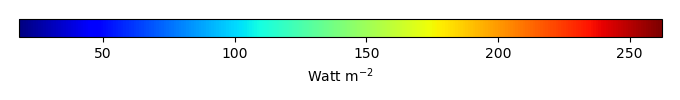MODEL MEANBIAS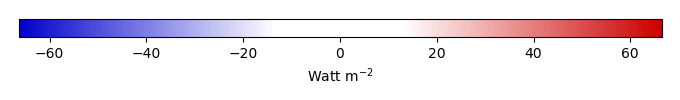BIAS SCORERMSE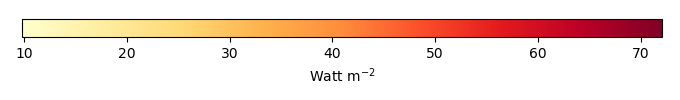RMSE SCOREBENCHMARK INTERANNUAL VARIABILITY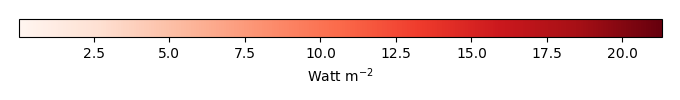MODEL INTERANNUAL VARIABILITYINTERANNUAL VARIABILITY SCOREBENCHMARK MAX MONTHMODEL MAX MONTHDIFFERENCE IN MAX MONTH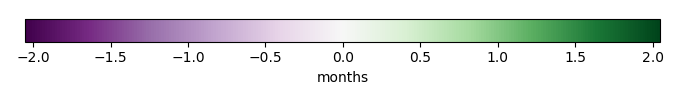SEASONAL CYCLE SCORESPATIAL TAYLOR DIAGRAMMODEL COLORS# Spatially integrated regional mean

MODEL COLORSREGIONAL MEANANNUAL CYCLEMONTHLY ANOMALYANNUAL CYCLE# All Models

BenchmarkCRUNCEPv7GSWP3v1WATCH# Data Information

creation_date: Tue Jul 1 08:29:23 PDT 2014

source_file: This product is generated from monthly 1 degree GEWEX SRB Radiation observations